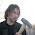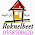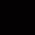## Monday, June 13, 2011

### More about Matrix to Euler angles conversion

I previously posted the algorithm to convert rotation matrix to arbitrarily ordered Euler angles.
It solved two problems of the trivial conversion method in Matrix and Quaternion FAQ:
• Use arbitrarily ordered axes (like ZXY) in addition to XYZ
• Remove the discontinuity happening at Ax = +/- 90 degrees
Meanwhile I discovered two remaining problems with my implementation:
• The algorithm always return the minimum set of absolute Euler angles (closest to {0;0;0}, as defined by the sum of squares of the angles) from the two alternatives. Often it is desirable to get the set of angles that are closest not to identity {0;0;0} but to some predefined rotation {Rx;Ry;Rz}.
• When gimbal lock occured, the X rotation (or corresponding rotation if axes were reordered) was set to zero. This may not be the best solution, if we need the Euler angles to be as close to some specific set of values as possible.
The problem surfaces if we want to describe animations by Euler angles to allow easy adjustments by IK solvers (and have joint constraints defined) and interpolate between keyframes. In that case we absolutely have to keep the angles of adjacent keyframes as similar as possible - because although the alternate sets of angles make identical matrices, interpolations between them generally do not.

Stable conversio of matrix to Euler angles closest to given set

The matrices have row-major order, so they have to be transposed for OpenGL.

Base is a set of three angles {Bx;By;Bz} that will be used as reference while determining the best set of resulting angles.

```void
Matrix3x4f::getEulerAngles (f32 angles[], const f32 base[]) const
{
float aX, aY, aZ;
float aX2, aY2, aZ2;

aY = asin (c);
aY2 = (aY >= 0) ? M_PI_F - aY : -M_PI_F - aY;
if (fabs (c) < 0.99999f) {
// Over 0.25 degrees from right angle
float C = cos (aY);
float C2 = cos (aY2);
float trX = c / C;
float trY = -c / C;
aX = atan2 (trY, trX);
aX2 = atan2 (-c / C2, c / C2);
trX = c / C;
trY = -c / C;
aZ = atan2 (trY, trX);
aZ2 = atan2 (-c / C2, c / C2);
} else {
// Less than 0.25 degrees from right angle
// Gimbal lock occured
aX = aX2 = base;
// Keep X rotation the same as base
float Sx = sin (aX);
float Cx = cos (aX);
if (fabs (Cx) > fabs (Sx)) {
float Sz = (c + (Sx/Cx) * c) / (Sx*Sx/Cx + Cx);
aZ = aZ2 = asin (Sz);
} else {
float Sz = (c + (Cx/Sx) * c) / (Cx*Cx/Sx + Sx);
aZ = aZ2 = asin (Sz);
}
}

float d1X = clamp_pi (aX - base);
float d1Y = clamp_pi (aY - base);
float d1Z = clamp_pi (aZ - base);
float d2X = clamp_pi (aX2 - base);
float d2Y = clamp_pi (aY2 - base);
float d2Z = clamp_pi (aZ2 - base);

if ((d1X * d1X + d1Y * d1Y + d1Z * d1Z) < (d2X * d2X + d2Y * d2Y + d2Z * d2Z)) {
angles[X] = aX;
angles[Y] = aY;
angles[Z] = aZ;
} else {
angles[X] = aX2;
angles[Y] = aY2;
angles[Z] = aZ2;
}
}```

If aY is close to +/- 90 degrees (gimbal lock occured) we want to set aX to the base value instead of setting it to zero.
Also we calculate the differences of both alternate sets of angles from base and choose the final set based on the squared sum of those differences.
Clamp_pi simply converts angle to -PI...PI range.

Conversion of matrix to arbitrarily ordered Eular angles

The matrices have row-major order, so they have to be transposed for OpenGL.

Order is array of three integers, determining the order of rotations ({0;1;2} is XYZ, {2;0;1} is ZXY).
Base values should be ordered according to order.

```void
Matrix3x4f::getEulerAngles (f32 angles[], const int order[], const f32 base[]) const
{
float sign = ((order == (order + 1)) || (order == (order + 1))) ? 1.0f : -1.0f;
// Permute matrix to switched axes space
Elea::Matrix3x4f rs;
for (int row = 0; row < 3; row++) {
for (int col = 0; col < 3; col++) {
rs[4 * row + col] = c[4 * order[row] + order[col]];
}
}
rs *= sign;
rs *= sign;
rs *= sign;
rs *= sign;
// New base
float nbase;
for (int i = 0; i < 3; i++) nbase[i] = base[i];
nbase *= sign;
// Decompose to Euler angles
rs.getEulerAngles (angles, nbase);
angles *= sign;
}
```

The trick is to permute the rotation matrix in such way, that the XYZ angles of new matrix are properly ordered decomposition angles of the original matrix and then do the trivial decomposition.
Hopefully this time the algorithm is final ;-)

Have fun!

1.Hi, what is c in:
+++++++++++++++++++
aY = asin (c);
+++++++++++++++++++

can you determine the final angle with a function like this:
---------------------------------
function cube.eulerAngle (factor )
--------------------------------------------------------------------------------
local heading = this.rotationY ( ) --theta
local attitude= this.rotationZ ( ) --phi
local bank = this.rotationX ( ) --psi

local c2 = math.cos(attitude/2)
local c3 = math.cos(bank/2)
local s2 = math.sin(attitude/2)
local s3 = math.sin(bank/2)

local angle = 2 * math.acos(c1*c2*c3 - s1*s2*s3)
local x = s1 * s2 * c3 +c1 * c2 * s3
local y = s1 * c2 * c3 +c1 * s2 * s3
local z = c1 * s2 * c3 -s1 * c2 * s3
x,y,z = math.vectorNormalize ( x,y,z )
object.rotateToAxisAngle ( this.getObject ( ),x,y,z, angle, object.kGlobalSpace,factor )
end

--------------------------------------------------------------------------------

for a discontinuity happening at Ax = +/- 90 degrees.
I want to rotate +-Y(90º) ,then +-X(90º).
Can you help me with this?
thanks!

2.Thanks for the article, Lauris! Everything makes sense to me except how you calculate the Z angle in your gimbal scenario. Could you write more about how you worked out the equations for
float Sz = (c + (Sx/Cx) * c) / (Sx*Sx/Cx + Cx);
and
float Sz = (c + (Cx/Sx) * c) / (Cx*Cx/Sx + Sx);

In that part of the loop, cos(y) is quickly approaching 0, so your c, c, c, and c elements are all approaching 0. Why would you solve c in terms of c, and wouldn't you also need to use c (or aY) to solve for sin(z)? I'm working through the math, and will possibly solve the equations in terms of something like c,c, and c, but my final equations have a lot more operations than yours do so I'm curious as to what I'm missing.

Thanks!
Michael

3.Oops, sorry, thank you for finding a mistake!
The correct formulas are:
Sz = (c + (Sx/Cx) * c) / (Sx*Sx/Cx+Cx);
Sz = (c + (Cx/Sx) * c) / (Cx*Cx/Sx+Sx);

The solving is basically following (for the case when Sx <> 0)
1. C = BDE+AF
1->3. E = (C-AF)/BD
4+2->5. F = C/B + AD(C-AF)/BBD
5->6. F = BC/BB + (AC - AAF)/BB
6->7. F = (BC + AC)/BB - AAF/BB
7->8. F(1+AA/BB) = (BC + AC)/BB
8->9. F(B+AA/B) = (BC + AC)/B B=/=0
9->10. F(B+AA/B) = C + AC/B

And similarly for the other acse (replace C and C while solving).

4.ما تهتم به شركة كشف تسربات المياه بالرياض هو البحث عن كيفة علاج مشاكل تسريبات المياه التي تطرأ علي المكان فجأة بواسطة اجهزة الكشف الحدية التي تستخدمها شركة كشف تسربات بالرياض والتي تسعي للوصول الي افضل النتائج المثالية القادرة علي حل هذه المشكلة بدون تدمير فالاعتماد علي الاساليب الحديثة يساعدكم في الحصول علي نتيجة مثالية في مصلحة العميل فنحن لا نكتفي بتقديم هذه الاعمال في مدينة الرياض فقط بلا لدينا الفنين المتميزة الذي يقدمون شركة كشف تسربات المياه بالدمام التي تعمل علي حل مشكلة البيت بدون الاعتماد علي ا اساليب تقليدية التي تستخدما بعض مقدمي خدمة شركة كشف تسربات بالدمام فلا تتكايل بشأن هذا العمل بالذات لانه يحل لك الكثير من المشاكل

5.العاب بنات يحتوي موقعنا على تشكيلة من العاب تلبيس بنات متجددة باستمرار وكل مايتعلق بصنف العاب بنات تلبيس ومكياج والعاب طبخ ومرحبا بكم في العاب تلبيس
العاب بنات - العاب تلبيس
العاب طبخ al3ab-banat01

6.Thank you for sharing! I hope you will continue to have great articles like this to share with everyone!

7.8.we have provide the best ppc service in Gurgaon.
ppc company in gurgaon
website designing company in Gurgaon

9.